# The three 4-flows conjecture

 Importance: Medium ✭✭
 Author(s): DeVos, Matt
 Subject: Graph Theory » Coloring » » Nowhere-zero flows
 Keywords: nowhere-zero flow
 Prize: bottle of wine (DeVos)
 Posted by: mdevos on: March 7th, 2007
Conjecture   For every graphwith no bridge, there exist three disjoint sets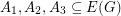withso that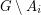has a nowhere-zero 4-flow for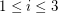.

A graphhas a nowhere-zero 4-flow if and only if there exist disjoint setswithso that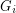has a nowhere-zero 2-flow for. Thus, the above conjecture is true with room to spare for such graphs. Since every 4-edge-connected graph and every 3-edge-colorable cubic graph has a nowhere-zero 4-flow, this conjecture is automatically true for these families. As with the 5-flow conjecture or the cycle double cover conjecture, establishing this conjecture comes down to proving it for cubic graphs which are not 3-edge-colorable.

This conjecture is a consequence of the Petersen coloring conjecture, and it implies the Orientable cycle four cover conjecture. The latter implication follows immediately from the fact that every graph with a nowhere-zero 4-flow has an orientable cycle double cover. Actually, it is possible that for every graphwith no cut-edge, there exist disjoint sets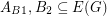with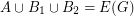and so thatandhave nowhere-zero 3-flows and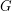has a nowhere-zero 2-flow. The Petersen graph has such a decomposition (and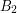should be alternate edges of some 8-circuit) and so does every graph with a nowhere-zero 4-flow. If this stronger statement is true, then it would imply the oriented eight cycle four cover conjecture.

## Bibliography

[J] F. Jaeger, On circular flows in graphs. Finite and infinite sets, Vol. I, II (Eger, 1981), 391--402, Colloq. Math. Soc. János Bolyai, 37, North-Holland, Amsterdam, 1984.. MathSciNet

* indicates original appearance(s) of problem.